# KDE plots for predicted probabilities in python

So I have previously written about two plots post binary prediction models – calibration plots and ROC curves. One addition to these I am going to show are kernel density estimate plots, broken down by the observed value vs predicted value. One thing in particular I wanted to make these for is to showcase the distribution of the predicted probabilities themselves, which can be read off of the calibration chart, but is not as easy.

I have written about this some before – transforming KDE estimates from logistic to probability scale in R. I will be showing some of these plots in python using the seaborn library. It will be easier instead of transforming the KDE to use edge weighting statistics to get unbiased estimates near the borders for the way the seaborn library is set up.

To follow along, you can download the data I will be using here. It is the predicted probabilities from the test set in the calibration plot blog post, predicting recidivism using several different models.

First to start, I load my python libraries and set my matplotlib theme (which is also inherited by seaborn charts).

Then I load in my data. To make it easier I am just working with the test set and several predicted probabilities from different models.

``````import pandas as pd
from scipy.stats import norm
import matplotlib
import matplotlib.pyplot as plt
import seaborn as sns

#####################
# My theme

andy_theme = {'axes.grid': True,
'grid.linestyle': '--',
'legend.framealpha': 1,
'legend.facecolor': 'white',
'legend.fontsize': 14,
'legend.title_fontsize': 16,
'xtick.labelsize': 14,
'ytick.labelsize': 14,
'axes.labelsize': 16,
'axes.titlesize': 20,
'figure.dpi': 100}

matplotlib.rcParams.update(andy_theme)
#####################``````

And here I am reading in the data (just have the CSV file in my directory where I started python).

``````################################################################
# Reading in the data with predicted probabilites
# Test from https://andrewpwheeler.com/2021/05/12/roc-and-calibration-plots-for-binary-predictions-in-python/
# https://www.dropbox.com/s/h9de3xxy1vy6xlk/PredProbs_TestCompas.csv?dl=0

print(pp_data.describe())
################################################################``````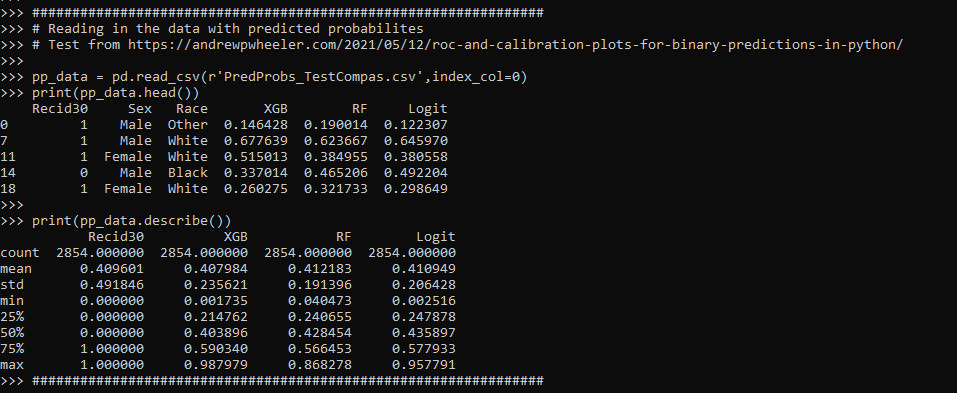So you can see this data has the observed outcome `Recid30` – recidivism after 30 days (although again this is the test dataset). And then it also has the predicted probability for three different models (XGBoost, RandomForest, and Logit), and then demographic breakdowns for sex and race.

The plot I am interested in seeing is a KDE estimate for the probabilities, broken down by the observed 0/1 for recidivism. Here is the default graph using seaborn:

``````# Original KDE plot by 0/1
sns.kdeplot(data=pp_data, x="Logit", hue="Recid30",
common_norm=False, bw_method=0.15)``````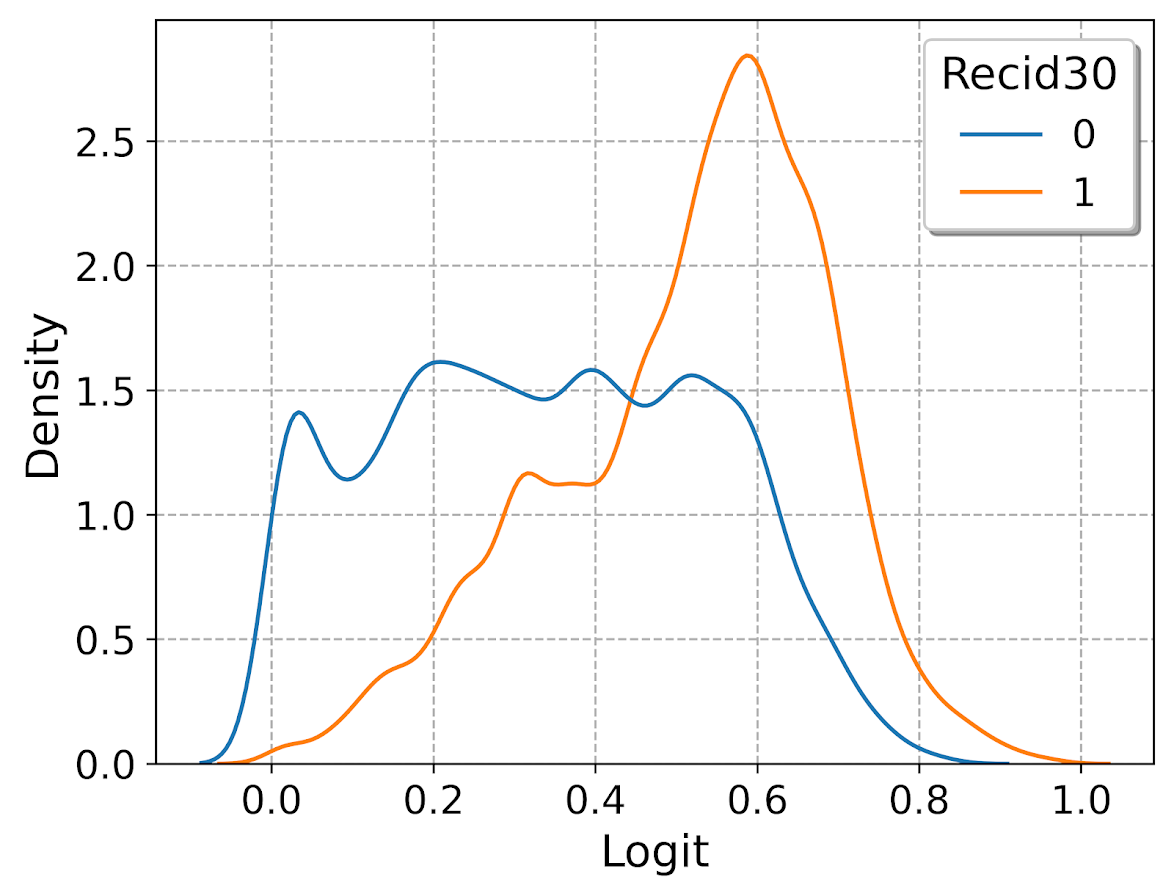One problem you can see with this plot though is that the KDE estimates are smoothed beyond the data. You cannot have a predicted probability below 0 or above 1. Because we are using a gaussian kernel, we can just reweight observations that are close to the edge, and then clip the KDE estimate. So a predicted probability of 0 would get a weight of 1/0.5 – so it gets double the weight. Note to do this correctly, you need to set the bandwidth the same for the seaborn kdeplot as well as the weights calculation – here 0.15.

``````# Weighting and clipping
# Amount of density below 0 & above 1
below0 = norm.cdf(x=0,loc=pp_data['Logit'],scale=0.15)
above1 = 1- norm.cdf(x=1,loc=pp_data['Logit'],scale=0.15)
pp_data['edgeweight'] = 1/ (1 - below0 - above1)

sns.kdeplot(data=pp_data, x="Logit", hue="Recid30",
common_norm=False, bw_method=0.15,
clip=(0,1), weights='edgeweight')``````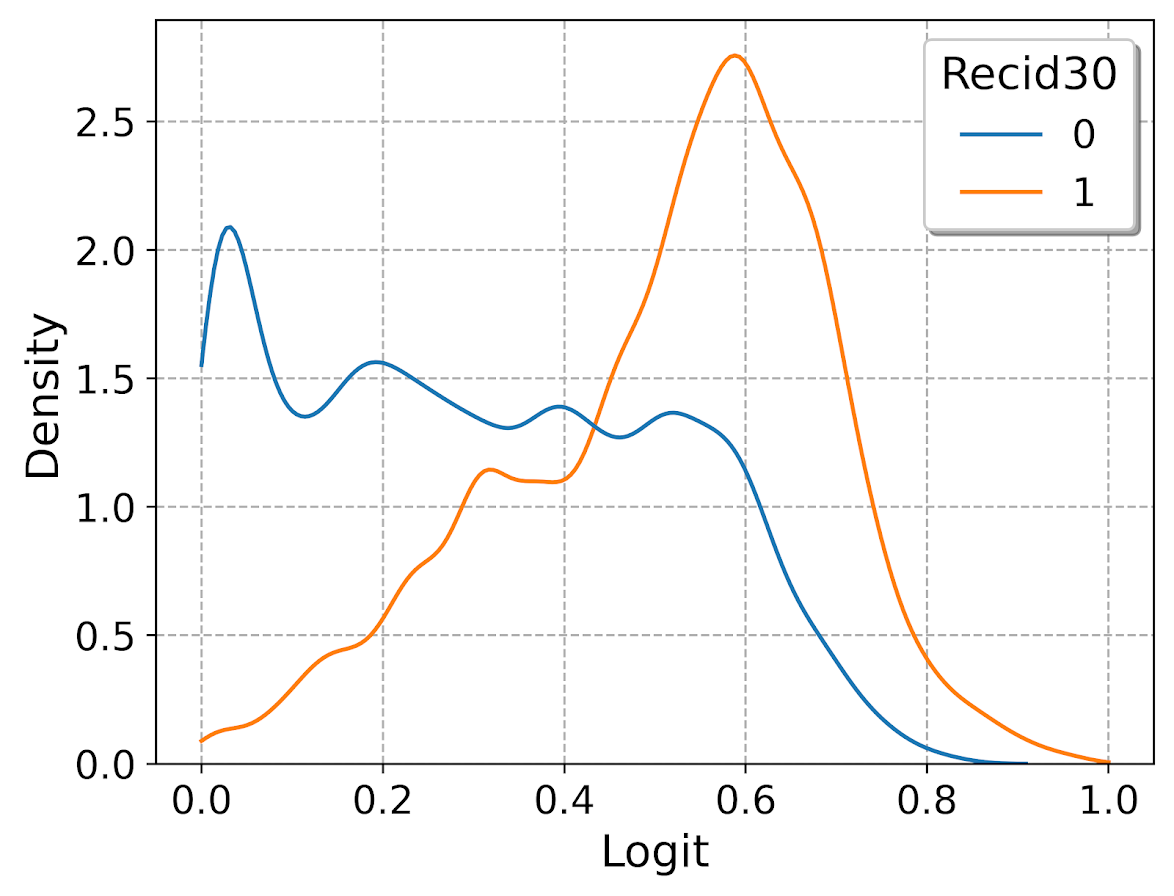This results in quite a dramatic difference, showing the model does a bit better than the original graph. The 0’s were well discriminated, so have many very low probabilities that were smoothed outside the legitimate range.

Another cool plot you can do that again shows calibration is to use seaborn’s fill option:

``````cum_plot = sns.kdeplot(data=pp_data, x="Logit", hue="Recid30",
common_norm=False, bw_method=0.15,
clip=(0,1), weights='edgeweight',
multiple="fill", legend=True)
cum_plot.legend_._set_loc(4) #via https://stackoverflow.com/a/64687202/604456``````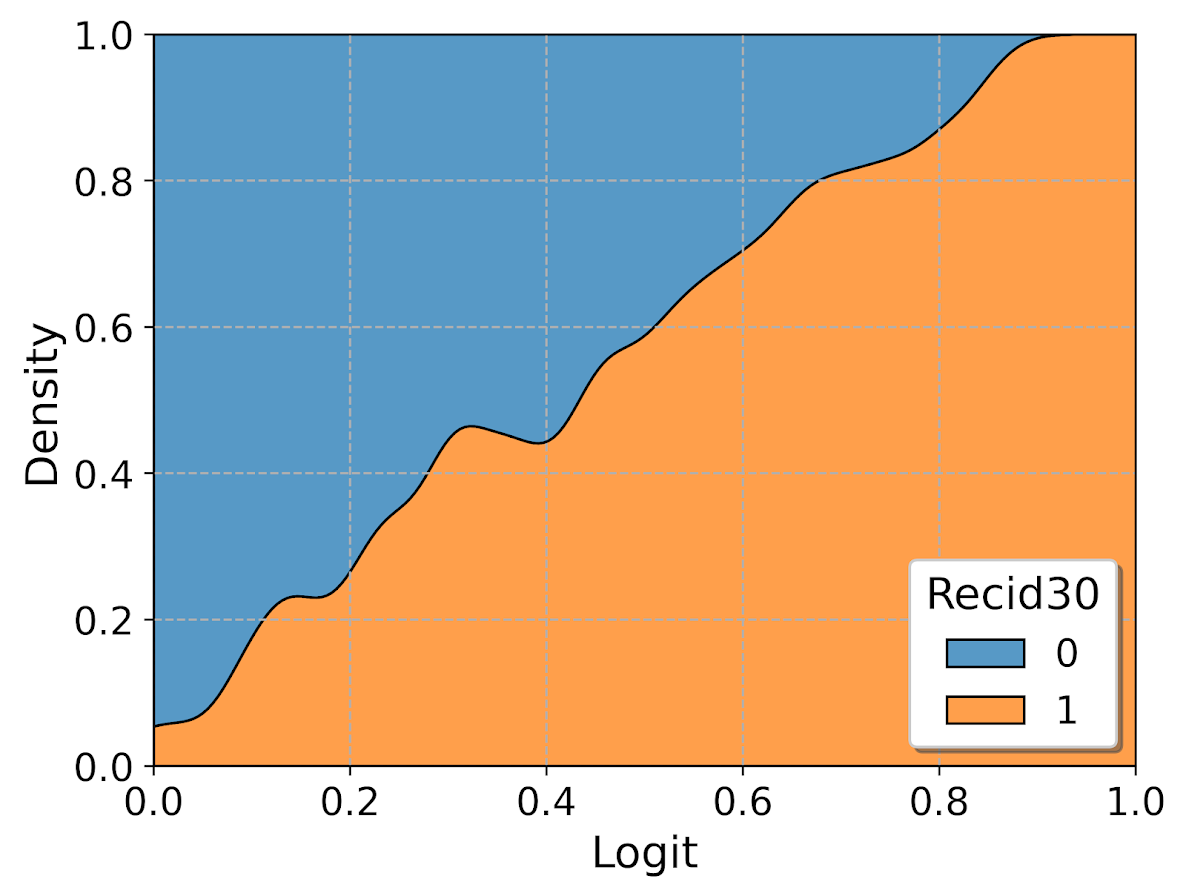As expected this shows an approximate straight line in the graph, e.g. 0.2 on the X axis should be around 0.2 for the orange area in the chart.

Next seaborn has another good function here, violin plots. Unfortunately you cannot pass a weight function here. But another option is to simply resample your data a large number of times, using the weights you provided earlier.

``````n = 1000000 #larger n will result in more accurate KDE
resamp_pp = pp_data.sample(n=n,replace=True, weights='edgeweight',random_state=10)

viol_sex = sns.violinplot(x="Sex", y="XGB", hue="Recid30",
data=resamp_pp, split=True, cut=0,
bw=0.15, inner=None,
scale='count', scale_hue=False)
viol_sex.legend_.set_bbox_to_anchor((0.65, 0.95))``````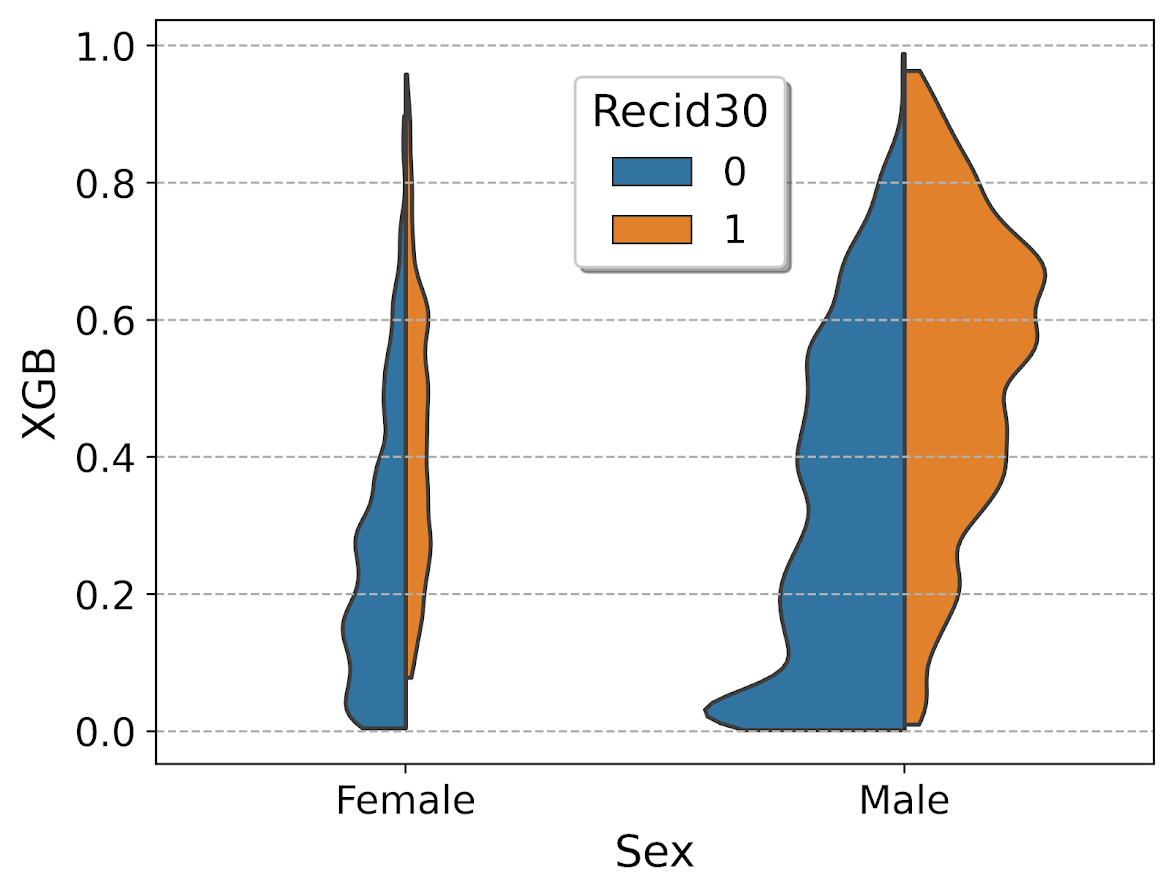So here you can see we have more males in the sample, and they have a larger high risk blob that was correctly identified. Females have a risk profile more spread out, although there is a small clump of basically 0 risk that the model identifies.

You can also generate the graph so the areas for the violin KDE’s are normalized, so in both the original and resampled data we have fewer females, and more black individuals.

``````# Values for Sex for orig/resampled
print(pp_data['Sex'].value_counts(normalize=True))
print(resamp_pp['Sex'].value_counts(normalize=True))

# Values for Race orig/resampled
print(pp_data['Race'].value_counts(normalize=True))
print(resamp_pp['Race'].value_counts(normalize=True))``````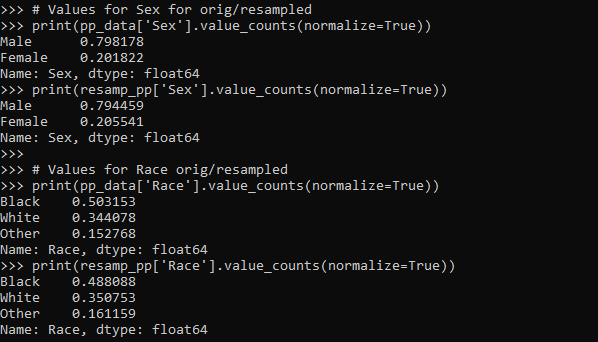But if we set `scale='area'` in the chart the violins are the same size:

``````viol_race = sns.violinplot(x="Race", y="XGB", hue="Recid30",
data=resamp_pp, split=True, cut=0,
bw=0.15, inner=None,
scale='area', scale_hue=True)
viol_race.legend_.set_bbox_to_anchor((0.81, 0.95))``````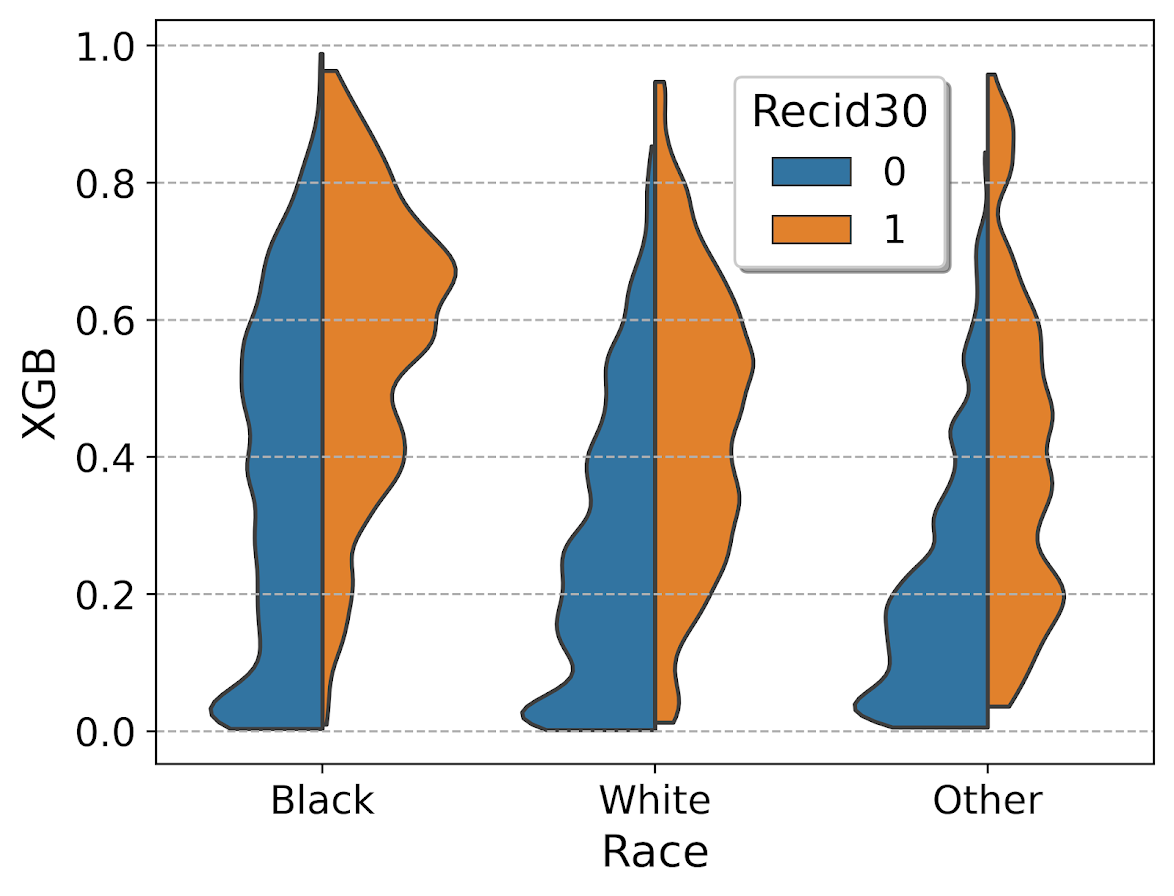I will have to see if I can make some time to contribute to seaborn to make it so you can pass in weights to the violinplot function.

# ROC and calibration plots for binary predictions in python

When doing binary prediction models, there are really two plots I want to see. One is the ROC curve (and associated area under the curve stat), and the other is a calibration plot. I have written a few helper functions to make these plots for multiple models and multiple subgroups, so figured I would share, binary plots python code. To illustrate their use, I will use the same Compas recidivism data I have used in the past, (CSV file here). So once you have downloaded those two files you can follow along with my subsequent code.

# Front Prep

First, I have downloaded the `binary_plots.py` file and the `PreppedCompas.csv` file to a particular folder on my machine, `D:\Dropbox\Dropbox\Documents\BLOG\binary_plots`. To import these functions, I append that path using `sys`, and change the working directory using `os`. The other packages are what I will be using the fit the models.

``````###############################################
# Front end prep

import pandas as pd
import numpy as np
from xgboost import XGBClassifier
from sklearn.ensemble import RandomForestClassifier
from sklearn.linear_model import LogisticRegression

import os
import sys

my_dir = r'D:\Dropbox\Dropbox\Documents\BLOG\binary_plots'
os.chdir(my_dir)

# Can append to path
sys.path.append(my_dir)
import binary_plots

np.random.seed(10) #setting the seed for the random
# split for train/test
###############################################``````

Next up I prepare the data, this is just boring stuff turning categorical variables into various dummies and making a train/test split for the data (which can be done in a variety of ways).

``````###############################################
# Prepping Compas Data

#For notes on data source, check out
#https://github.com/apwheele/ResearchDesign/tree/master/Week11_MachineLearning

#Preparing the variables I want
recid_prep = recid[['Recid30','CompScore.1','CompScore.2','CompScore.3',
recid_prep['Male'] = 1*(recid['sex'] == "Male")
recid_prep['Fel'] = 1*(recid['c_charge_degree'] == "F")
recid_prep['Mis'] = 1*(recid['c_charge_degree'] == "M")

print( recid['race'].value_counts() )
dum_race = pd.get_dummies(recid['race'])
# White for reference category
for d in list(dum_race):
if d != 'Caucasion':
recid_prep[d] = dum_race[d]

print( recid['marital_status'].value_counts() )
dum_mar = pd.get_dummies(recid['marital_status'])
recid_prep['Single'] = dum_mar['Single']
recid_prep['Married'] = dum_mar['Married'] + dum_mar['Significant Other']
# reference category is separated/unknown/widowed

#Now generating train and test set
recid_prep['Train'] = np.random.binomial(1,0.75,len(recid_prep))
recid_train = recid_prep[recid_prep['Train'] == 1].copy()
recid_test = recid_prep[recid_prep['Train'] == 0].copy()

#Independant variables
ind_vars = ['CompScore.1','CompScore.2','CompScore.3',
'African-American','Asian','Hispanic','Native American','Other',
'Single','Married']

# Dependent variable
y_var = 'Recid30'
###############################################``````

Next, the sklearn library makes it quite easy to fit a set of multiple models. Most of the time I start with XGBoost, random forests, and a normal logistic model with no coefficient penalty. I just stuff the base model object in a dictionary, pipe in the same training data, and fit the models. Then I can add in the predicted probabilities from each model into the test dataset. (These plots I show you should only show on the test dataset, of course the data will be calibrated on the training dataset.)

``````###############################################
# Training three different models, Logit,
# Random Forest, and XGBoost

final_models = {}
final_models['XGB'] = XGBClassifier(n_estimators=100, max_depth=5)
final_models['RF'] = RandomForestClassifier(n_estimators=1000, max_depth=10, min_samples_split=50)
final_models['Logit'] = LogisticRegression(penalty='none', solver='newton-cg')

# Iterating over each model and fitting on train
for nm, mod in final_models.items():
mod.fit(recid_train[ind_vars], recid_train[y_var])

# Adding predicted probabilities back into test dataset
for nm, mod in final_models.items():
# Predicted probs out of sample
recid_test[nm] =  mod.predict_proba(recid_test[ind_vars])[:,1]
###############################################``````

This is fairly tiny data, so I don’t need to worry about how long this takes or run out of memory. I’d note you can do the same model, but different hyperparameters in this approach. Such as tinkering with the depth for tree based models is one I by default limit quite a bit.

# AUC Plots

First, my goto metric to see the utility of a particular binary prediction model is the AUC stat. This has one interpretation in terms of the concordance stat, an AUC of 0.7 means if you randomly picked a 0 case and a 1 case, the 1 case would have a higher value 70% of the time. So AUC is all about how well your prediction discriminates between the two classes.

So with my `binary_plots` function, you can generate an ROC curve for the test data for a single column of predictions as so:

``````# A single column
binary_plots.auc_plot(recid_test, y_var, ['Logit'], save_plot='AUC1.png')``````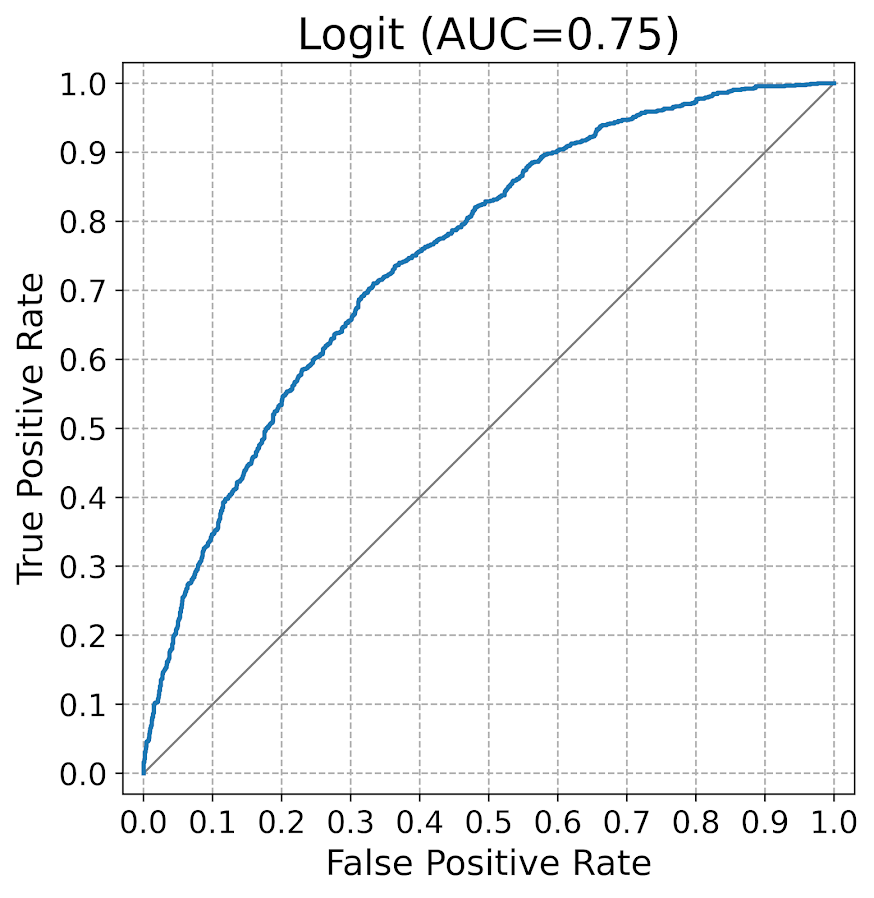As I have generated predictions for multiple models, I have also generated a similar graph, but stuff the AUC stats in the matplotlib legend:

``````# Multiple columns to show different models
pred_prob_cols = list(final_models.keys()) #variable names
binary_plots.auc_plot(recid_test, y_var, pred_prob_cols, save_plot='AUC2.png')``````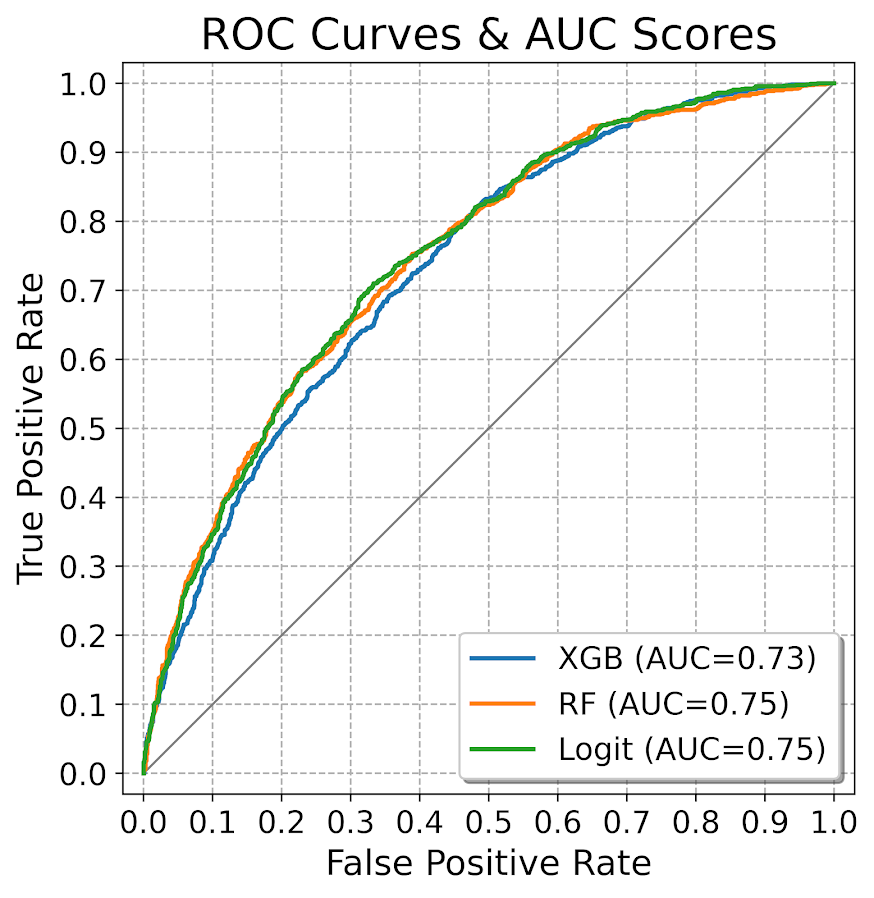It is also the case you want to do these plots for different subgroups of data. In recidivism research, we are often interested in sex and racial breakdowns. Here is the Logit model AUC broken down by Males (1) and Females (0).

``````# By subgroups in the data
binary_plots.auc_plot_long(recid_test, y_var, 'Logit', group='Male', save_plot='AUC3.png')``````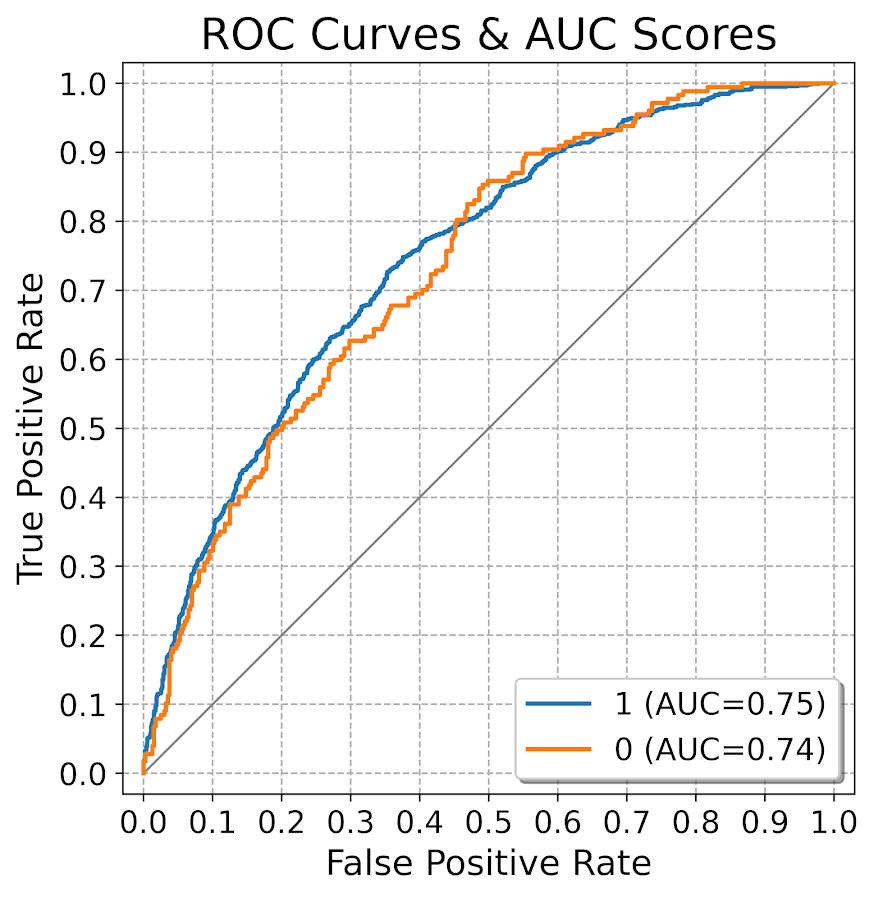So this pulls the labels from the data, but you can pass in strings to get nicer labels. And finally, I show how to put both of these together, both by models and by subgroups in the data. Subgroups are different panels, and you can pass in a fontsize moniker to make the legends smaller for each subplot, and a size for each subplot (they are squares).

``````# Lets make nicer variable names for Male/Females and Racial Groups
recid_test['Sex'] = recid_test['Male'].replace({0: 'Female', 1:'Male'})
recid_test['Race'] = recid[recid_prep['Train'] == 0]['race']
recid_test['Race'] = recid_test['Race'].replace({'Hispanic': 'Other', 'Asian':'Other', 'Native American':'Other', 'African-American':'Black', 'Caucasian':'White'})

# Now can do AUC plot by gender and model type
binary_plots.auc_plot_wide_group(recid_test, y_var, pred_prob_cols, 'Sex', size=4, leg_size='x-small', save_plot='AUC4.png')``````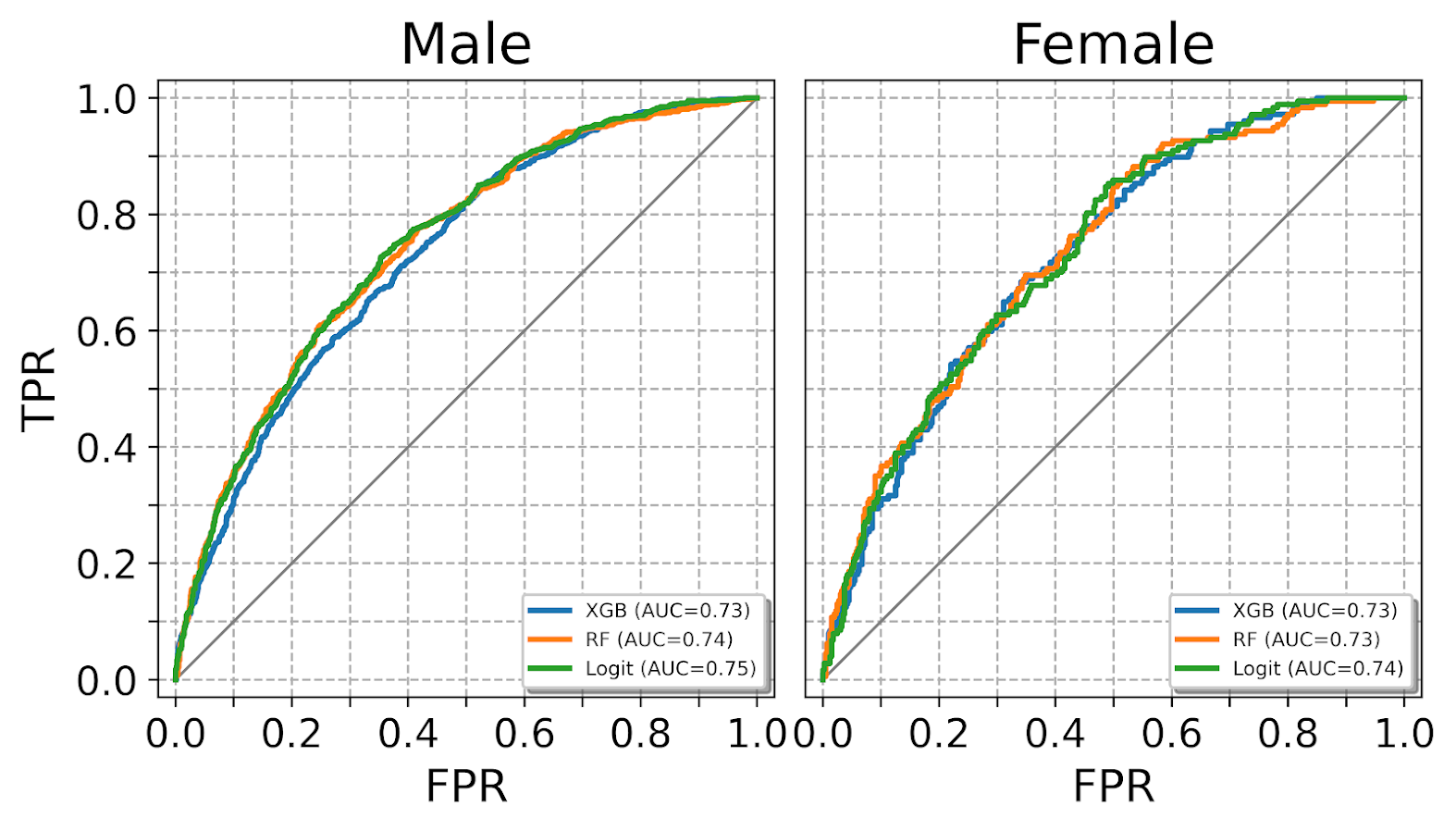The plots have a wrap function (default wrap at 3 columns), so you can plot as many subgroups as you want. Here is an example combing the sex and race categories: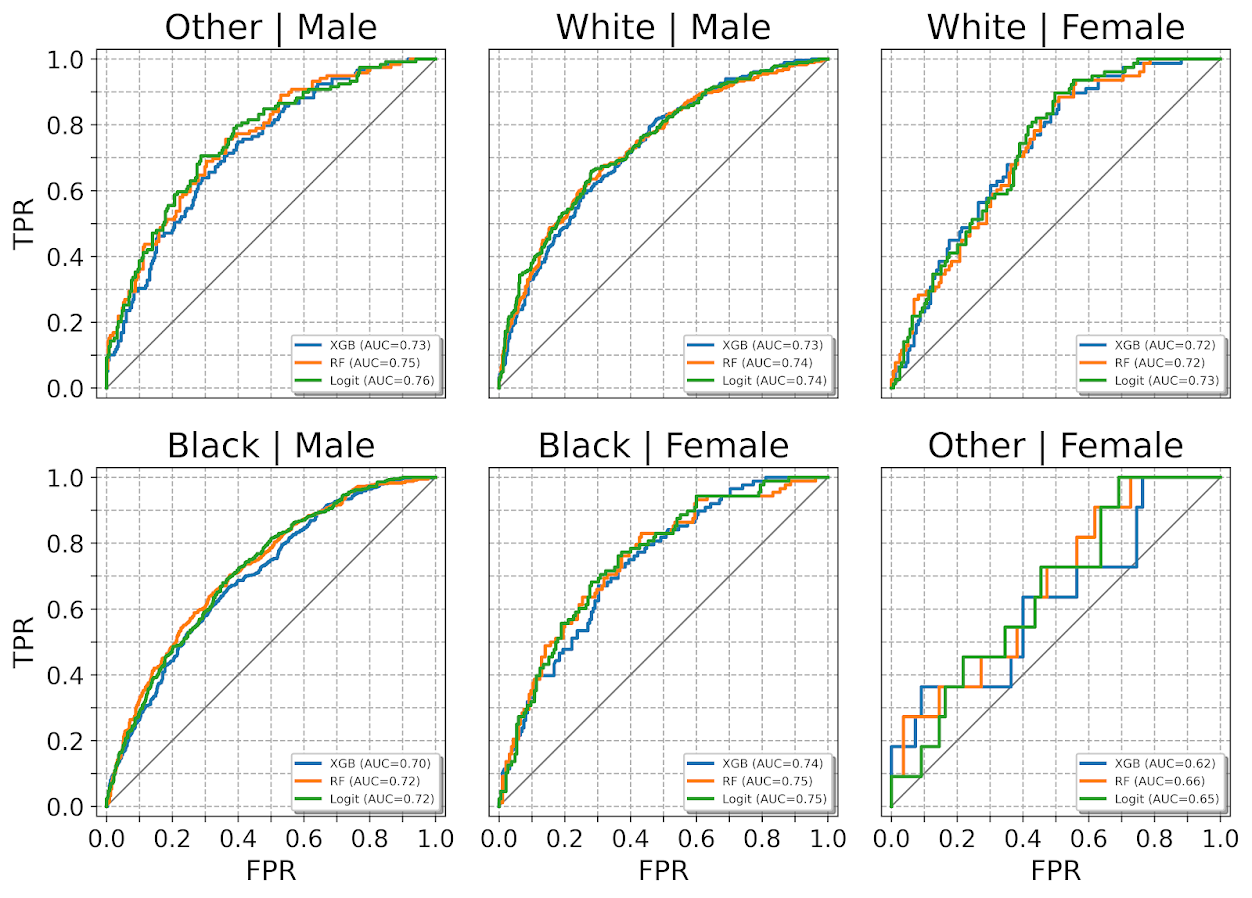One limitation to note in these plots, ROC plots are normalized in a way that the thresholds for each subgroup may not be at the same area of the plot (e.g. a FPR of 0.1 for one subgroup implies a predicted probability of 30%, whereas for another subgroup it implies a predicted probability of 40%).

ROC/AUC is definitely not a perfect stat, most of the time we are only interested in the far left hand side of the ROC curve (how well we can identify high risk cases without a ton of false positives). That is why I think drawing the curves are important – one model may have a higher AUC, but it is in an area of the curve not relevant for how you will use the predictions in practice. (For tree based models with limited depth and limited variables, it can produce flat areas in the ROC curve for example.)

But I find the ROC curve/AUC metric the most useful default for both absolute comparisons (how well is this model doing overall), as well as relative model comparisons (is Model A better than Model B).

Most models I work with I can get an AUC of 0.7 without much work, and once I get an AUC of 0.9 I am in the clearly diminishing returns category to tinkering with my model (this is true for both criminology related models I work with, as well as healthcare related models in my new job).

This is of course data dependent, and even an AUC of 0.9 is not necessarily good enough to use in practice (you need to do a cost-benefit type analysis given how you will use the predictions to figure that out).

# Calibration Charts

For those with a stat background, these calibration charts I show are a graphical equivalent of the Hosmer-Lemeshow test. I don’t bother conducting the Chi-square test, but visually I find them informative to not only see if an individual model is calibrated, but also to see the range of the predictions (my experience XGBoost will be more aggressive in the range of predicted probabilities, but is not always well calibrated).

So we have the same three types of set ups as with the ROC plots, a single predicted model:

``````# For single model
binary_plots.cal_data('XGB', y_var, recid_test, bins=60, plot=True, save_plot='Cal1.png')``````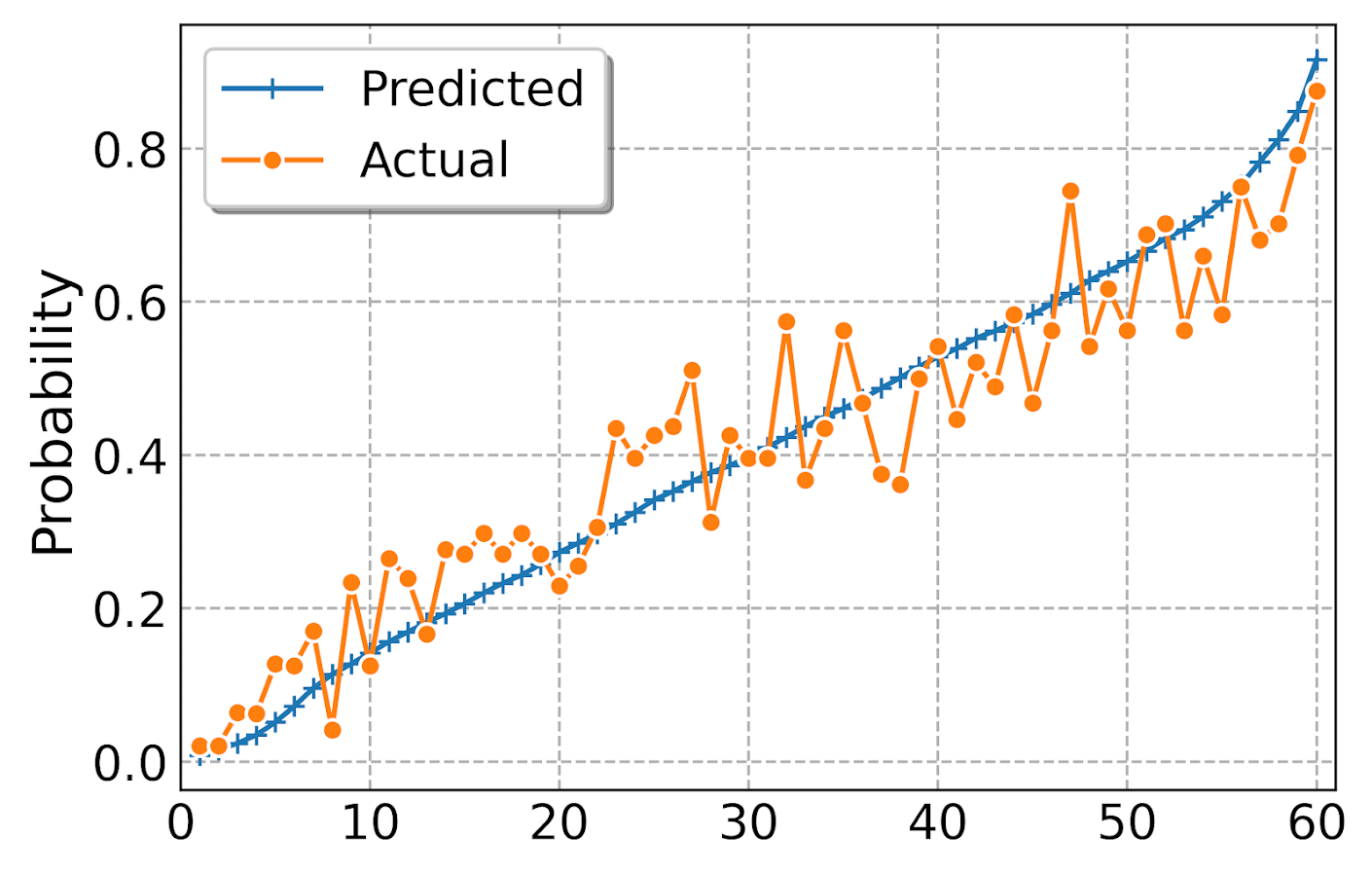For multiple models, I always do these on separate subplots, they would be too busy to superimpose. And because it is a single legend, I just build the data and use seaborn to do a nice small multiple. (All of these functions return the dataframe I use to build the final plot in long format.) The original plot was slightly noisy with 60 bins, so I reduce it to 30 bins here, but it is still slightly noisy (but each model is pretty well calibrated). XGBoost has a wider range of probabilities, random forests lowest bin is around 0.1 and max is below 0.8. Logit has lower probabilities but none above 0.8.

``````# For multiple models
binary_plots.cal_data_wide(pred_prob_cols, y_var, recid_test, bins=30, plot=True, save_plot='Cal2.png')``````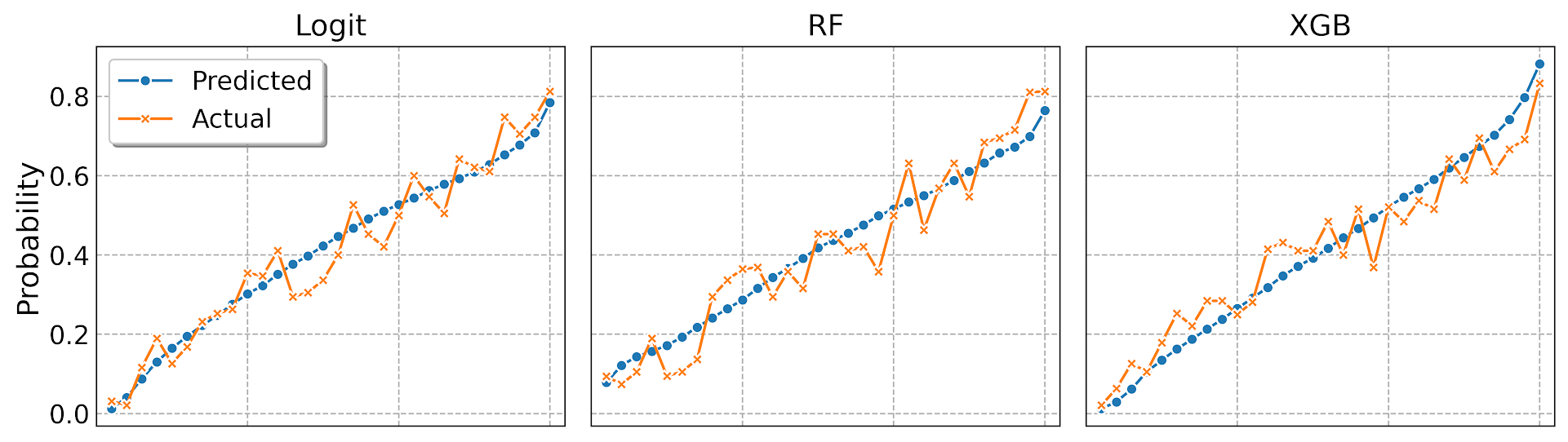For a single model, but by subgroups in the data. The smaller other race group is more noisy, but again each model appears to be approximately calibrated.

``````# For subgroups and one model
binary_plots.cal_data_group('XGB', y_var, 'Race', recid_test, bins=20, plot=True, save_plot='Cal3.png')``````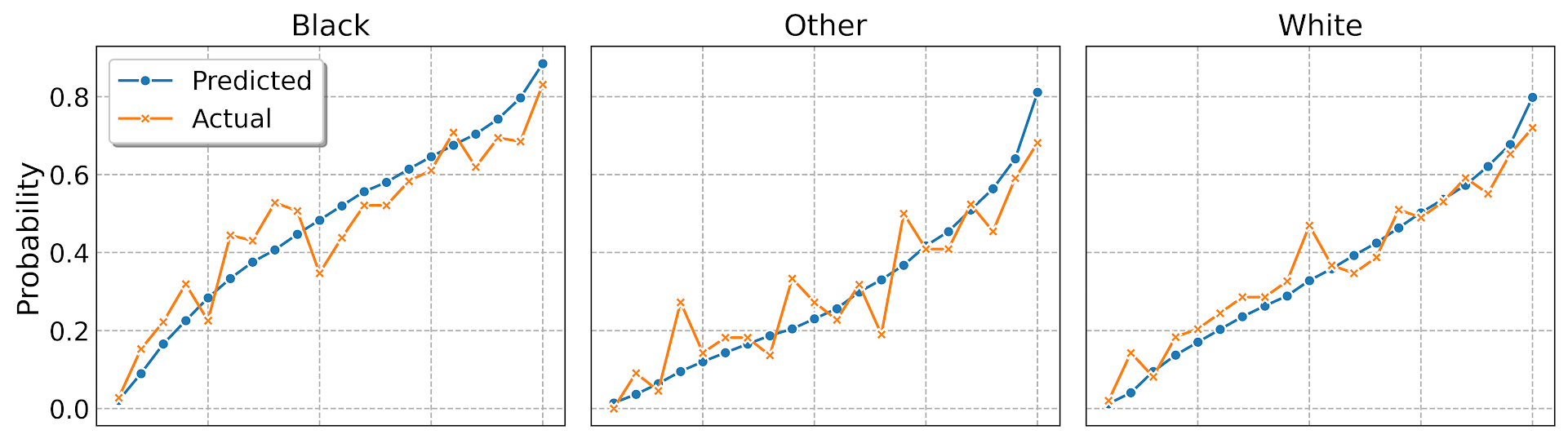And a combo of subgroup data and multiple models. Again the smaller subgroup Females appear more noisy, but all three models appear to be doing OK in this quick example.

``````# For subgroups and multiple models
binary_plots.cal_data_wide_group(pred_prob_cols, y_var, 'Sex', recid_test, bins=20, plot=True, save_plot='Cal4.png')``````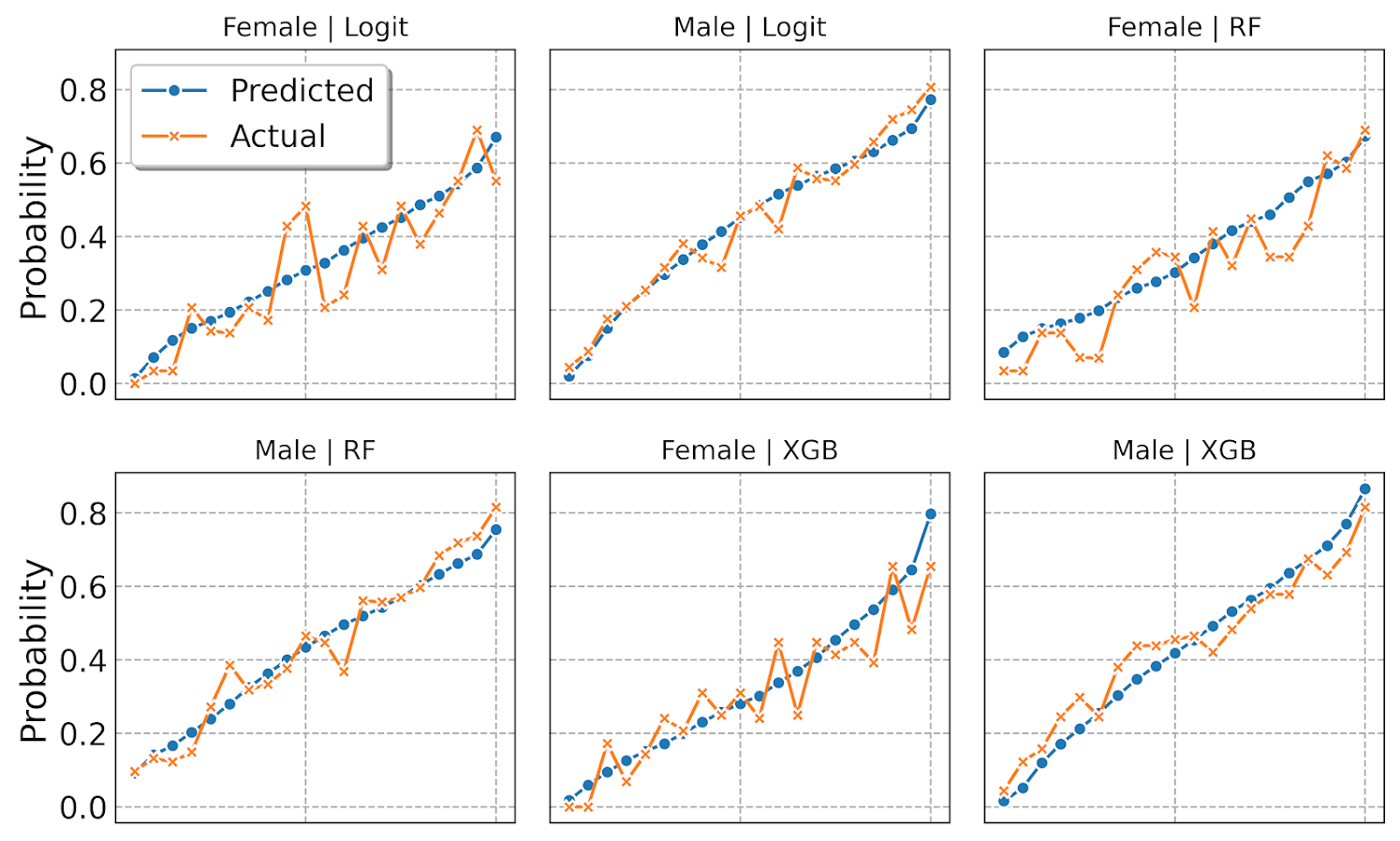Sometimes people don’t bin the data (Peter Austin likes to do a smoothed plot), but I find the binned data easier to follow and identify deviations above/below predicted probabilities. In real life you often have some fallout/dropoff if there is feedback between the model and how other people respond to the model (e.g. the observed is always 5% below the predicted).